# Ensemble/Boosting¶

## Ensemble Methods¶

• use an ensemble/group of hypotheses
• diversity important; ensemble of “yes-men” is useless
• get diverse hypotheses by using
• different data
• different algorithms
• different hyperparameters

Why?

• averaging reduces variance
• ensemble more stables than individual
• consider biased coin p(head) = 1/3
• variance on one flip = 1/3 - 1/9 = 2/9
• variance on average of 2 flips = 2/9 - 1/9 = 1/9
• averaging makes less mistakes
• consider 3 classifiers with accuracy 0.8, 0.7, and 0.7
• the probability that the majority vote is correct = (f1 correct, f2 correct, f3 wrong) + (f1 correct, f2 wrong, f3 correct) + (f1 wrong, f2 correct, f3 correct) + (f1 correct, f2 correct, f3 correct)
• = $$(.8*.7*.3) + (.8*.3*.3) + (.2*.7*.7) + (.8*.8*.7) \approx 0.82$$

### Creation¶

• use different training sets
• bootstrap example: pick m examples from labeled data with replacement
• cross-validation sampling
• reweight data (boosting, later)
• use different features
• random forests “hide” some features

### Prediction¶

• unweighted vote
• weighted vote
• pay more attention to better predictors
• filter examples; each level predicts or passes on

## Boosting¶

• “boosts” the preformance of another learning algorithm
• creates a set of classifiers and predicts with weighted vote
• use different distributions on sample to get diversity
• up-weight hard, down-weight easy examples
• note: ensembles used before to reduce variance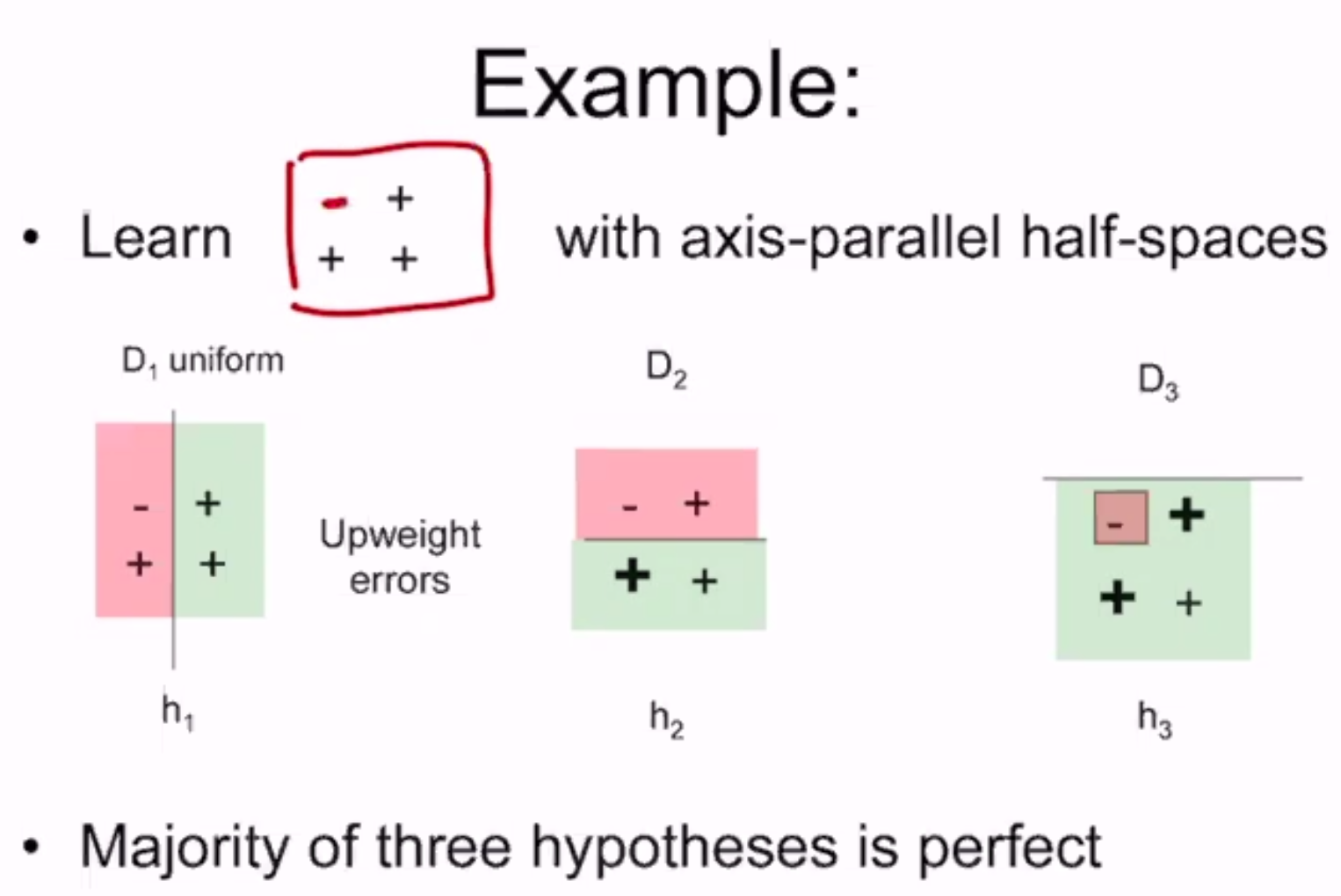If boosting is possible, then:

• can use fairly wild guesses to produce highly accurate predictions
• if you can learn “part way” you can learn “all the way”
• should be able to improve any learning algorithm
• for any learning problem:
• either can learn always with nearly perfect accuracy
• or there exist cases where cannot learn even slightly better than random guessing

Given a training sample S with labels +/-, and a learning algorithm L:

1. for t from 1 to T do
1. create distribution $$D_t$$ on $$S$$
2. call $$L$$ with $$D_t$$ on $$S$$ to get hypothesis $$h_t$$
1. i.e. $$\min \sum_n D_t(n) l(f(x_n), y_n)$$ where $$D_t(n)$$ is the weight of the sample
3. calculate weight $$\alpha_t$$ for $$h_t$$
2. final hypothesis is $$F(x) = \sum_t \alpha_t h_t(x)$$, or $$H(x)$$ = value with most weight

formally: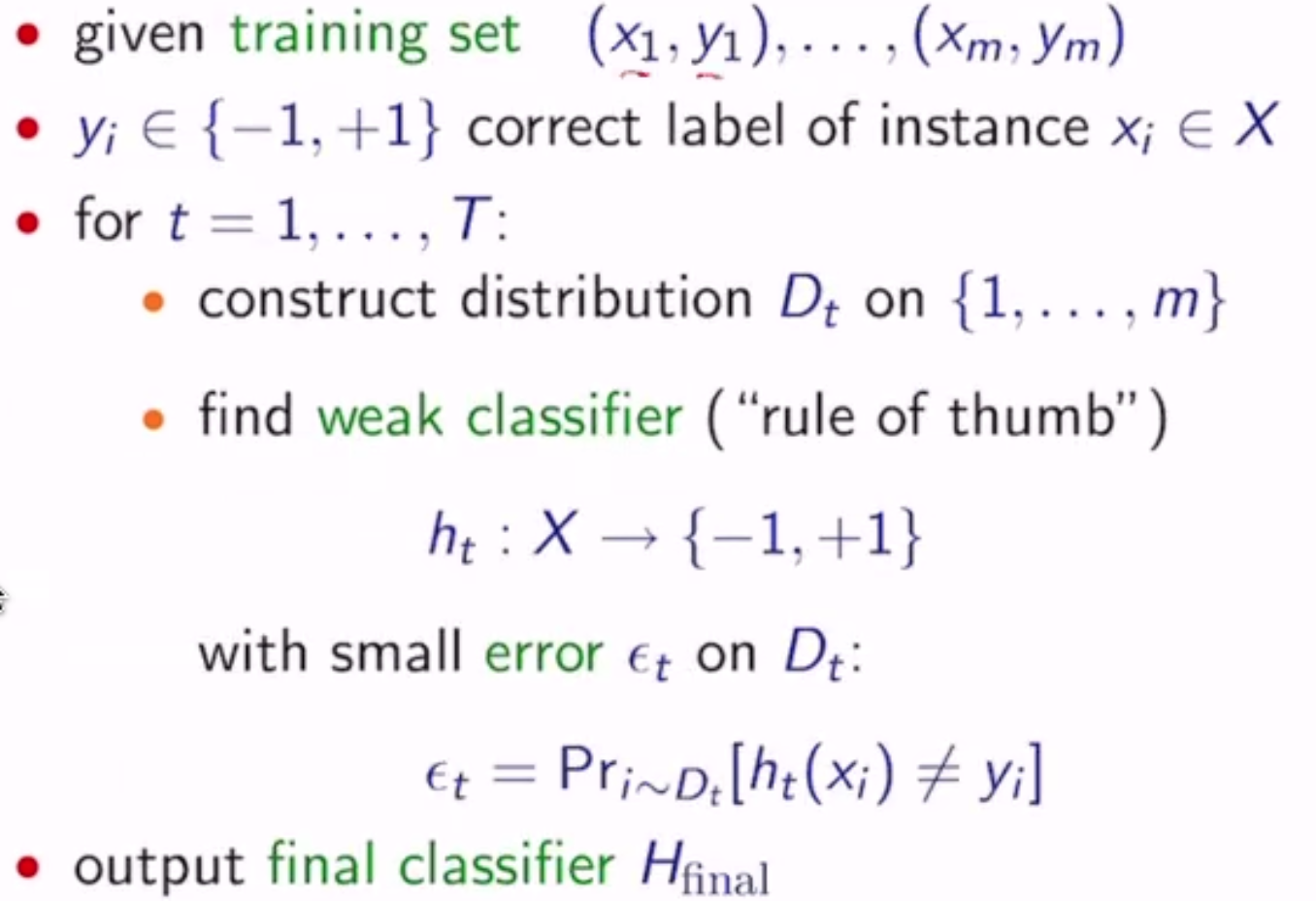So how do we pick $$D_t$$ and $$\alpha_t$$?

• $$D_1(i) = 1/m$$ - the weight assigned to $$(x_i, y_i)$$ at $$t=1$$
• given $$D_t$$ and $$h_t$$:
• $$D_{t+1}(i) = \frac{D_t(i)}{Z_t} \exp(-\alpha_t y_i h_t(x_i))$$
• if correct, increase weights by a factor > 1 (positive exponential)
• otherwise decrease by a factor < 1 (negative exponential)
• where $$Z_t$$ is a normalization factor
• where $$\alpha_t = \frac{1}{2} \ln (\frac{1-\epsilon_t}{\epsilon_t}) > 0$$
• $$H_{final}(x) = sign(\sum_t \alpha_t h_t(x))$$

Example: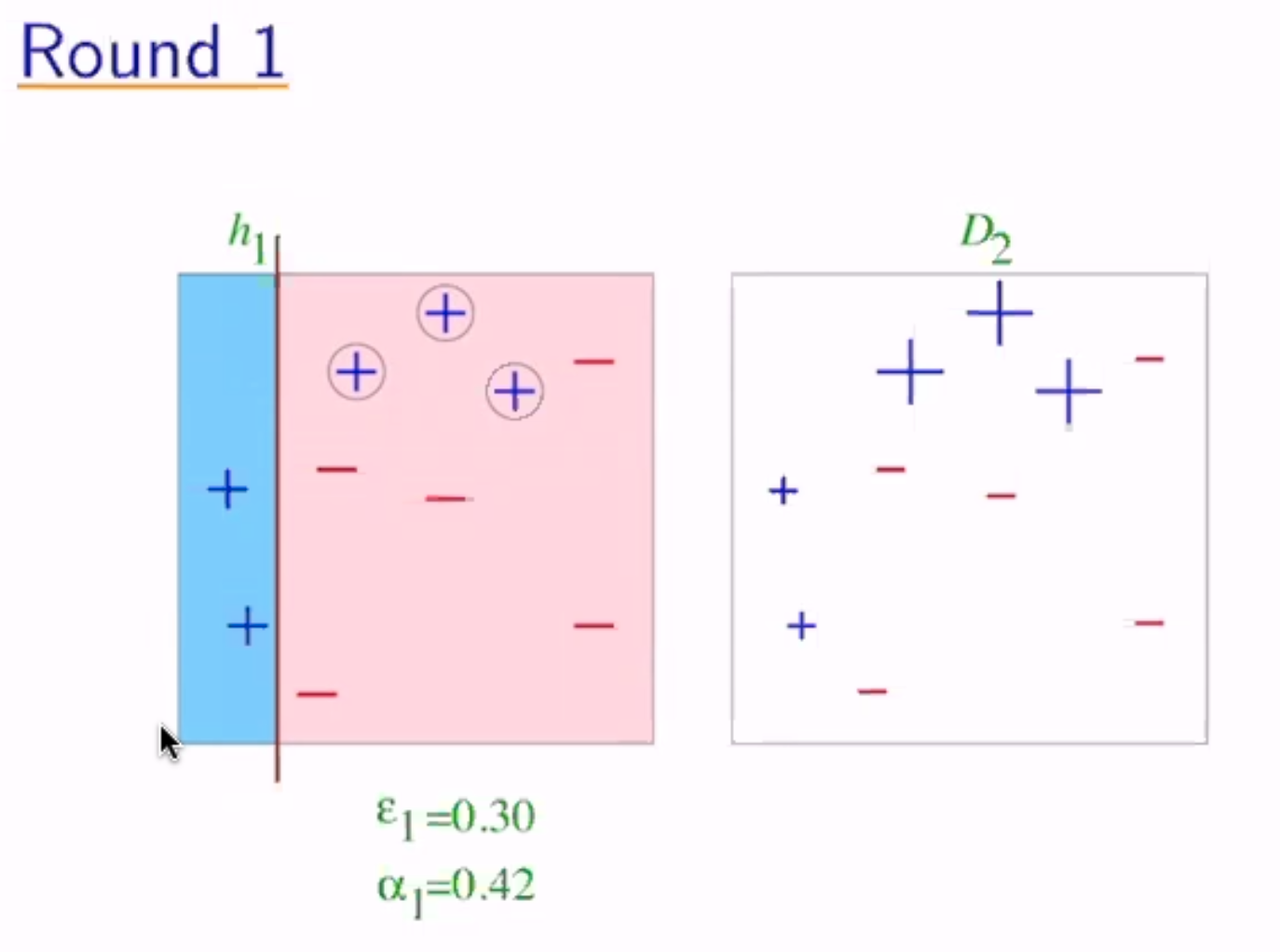now the weights become $$\frac{1}{10} e^{0.42}$$ for the misclassified and $$\frac{1}{10} e^{-0.42}$$ for the correct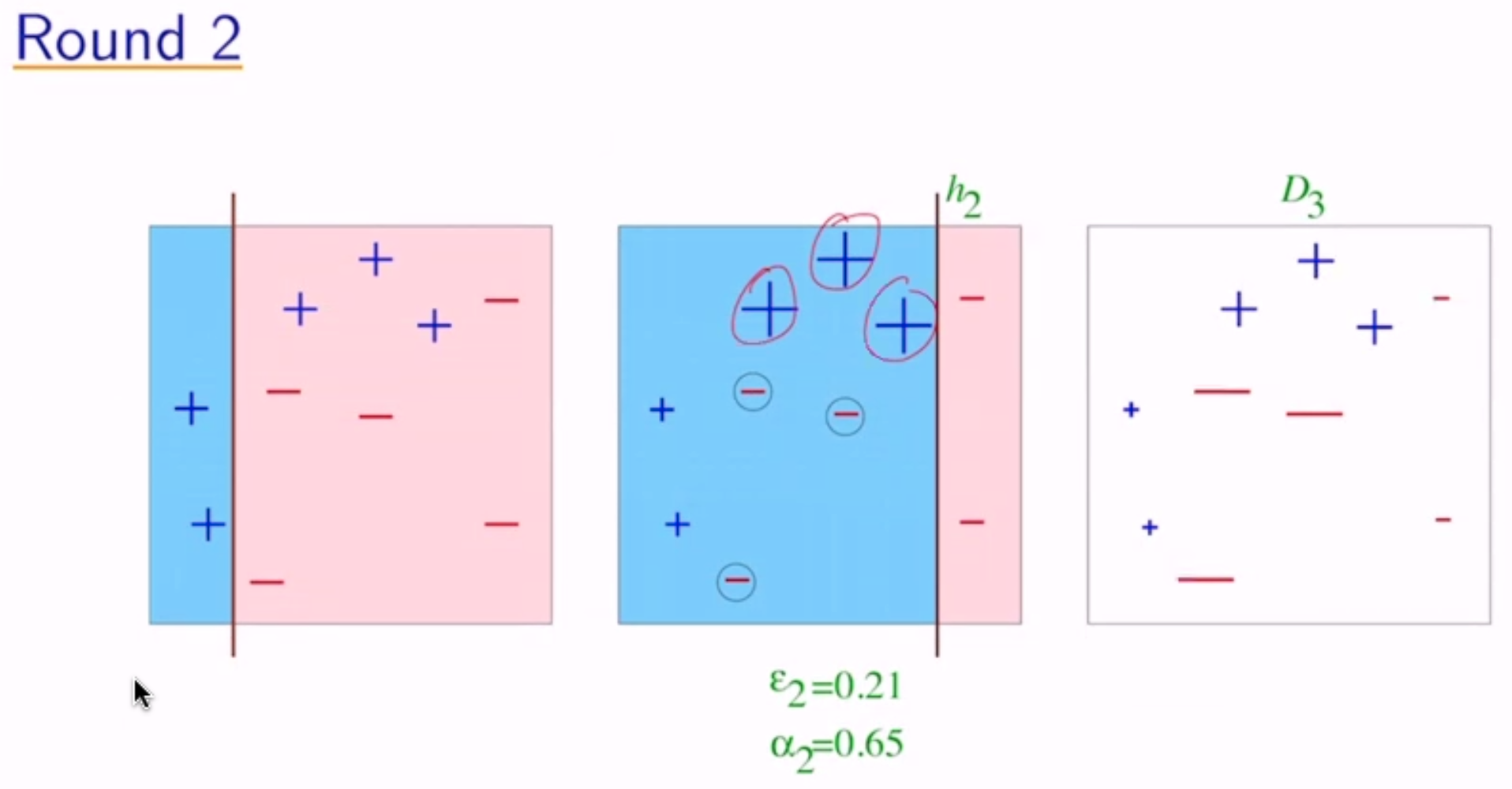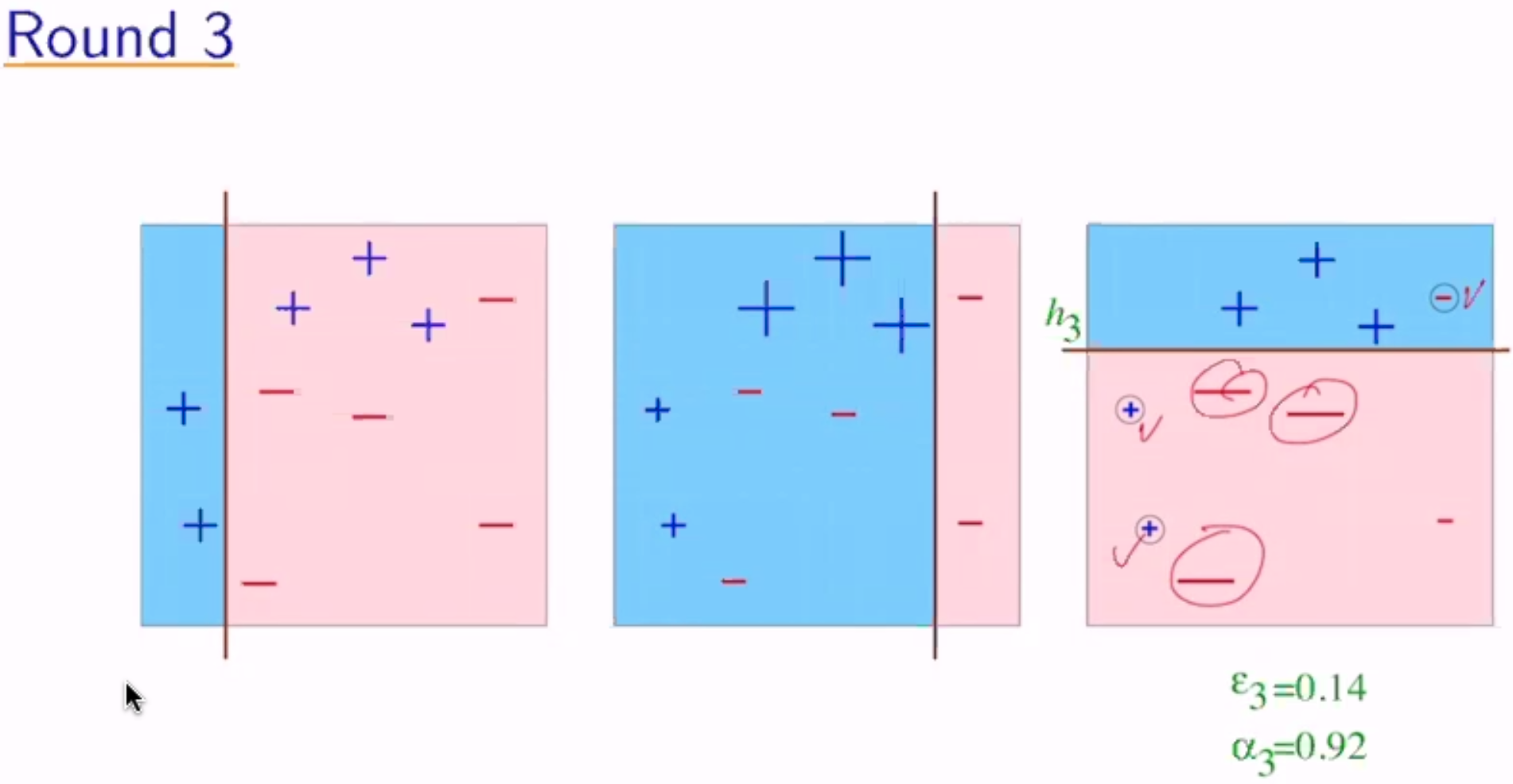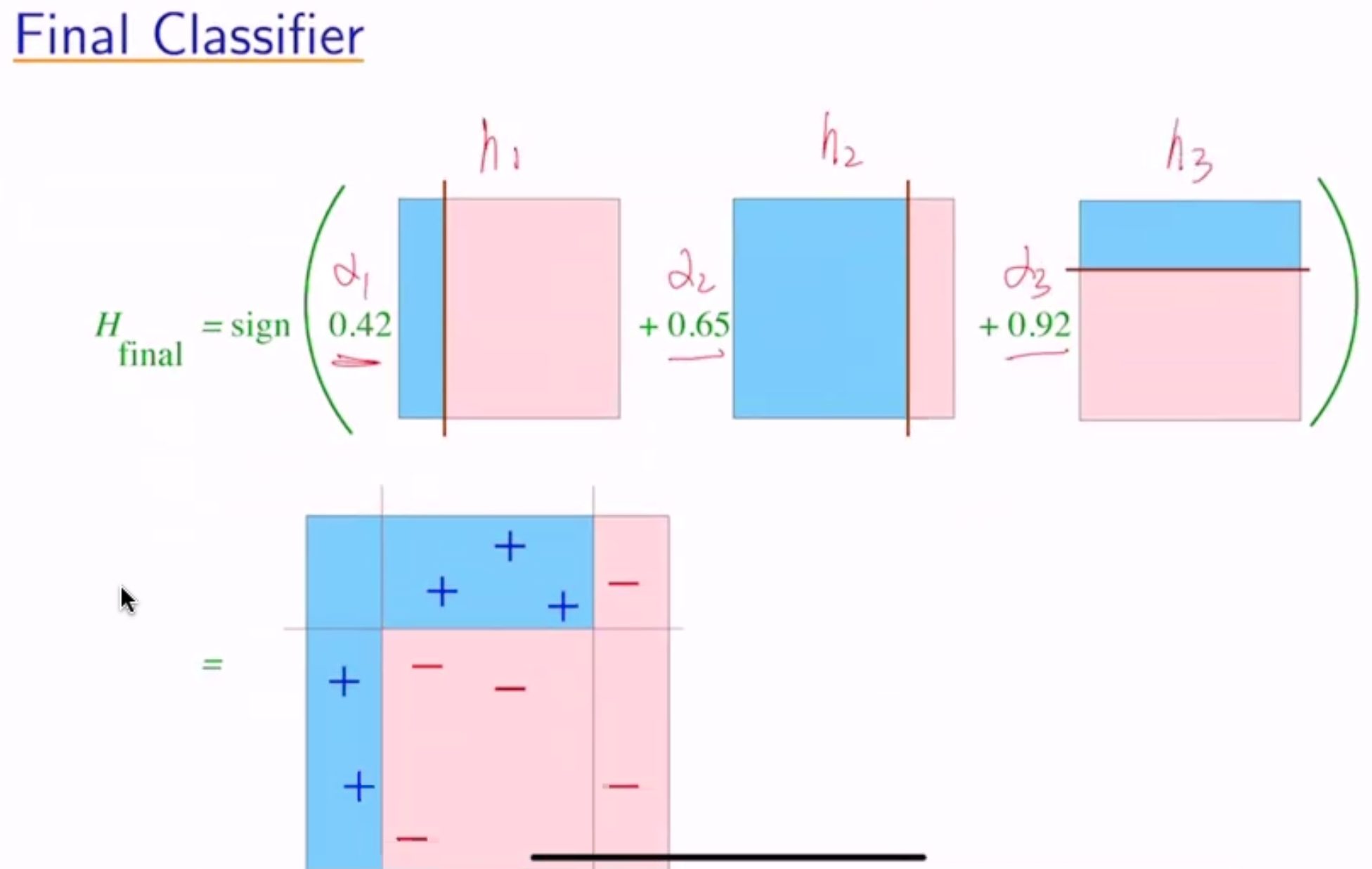### Analyzing Error¶

Thm: Write $$\epsilon_t$$ as $$1/2 - \gamma_t$$ - $$\gamma_t$$ = “edge” = how much better than random guessing. Then:

$\begin{split}\text{training error}(H_{final}) & \leq \prod_t [2 \sqrt{\epsilon_t (1-\epsilon_t)}] \\ & = \prod_t \sqrt{1-4\gamma_t^2} \\ & \leq \exp(-2\sum_t \gamma_t^2)\end{split}$

So if $$\forall t: \gamma_t \geq \gamma > 0$$, then $$\text{training error}(H_{final}) \leq e^{-2\gamma^2 T}$$

therefore, as $$T \to \infty$$, training error $$\to 0$$

### Proof¶

Let $$F(x) = \sum_t \alpha_t h_t(x) \to H_{final}(x) = sign(F(x))$$

Step 1: unwrapping recurrence

$\begin{split}D_{final}(i) & = \frac{1}{m} \frac{\exp(-y_i \sum_t \alpha_t h_t(x_i))}{\prod_t Z_t} \\ & = \frac{1}{m} \frac{\exp(-y_i F(x_i))}{\prod_t Z_t}\end{split}$

Step 2: training error $$(H_{final}) \leq \prod_t Z_t$$

$\begin{split}\text{training error}(H_{final}) & = \frac{1}{m} \sum_i & 1 & \text{ if } y_i \neq H_{final}(x_i) \\ & & 0 & \text{ otherwise} \\ & = \frac{1}{m} \sum_i & 1 & \text{ if } y_i F(x_i) \leq 0 \\ & & 0 & \text{ otherwise} \\ & \leq \frac{1}{m} \sum_t \exp(-y_i F(x_i)) \\ & = \sum_i D_{final}(i) \prod_t Z_t \\ & = \prod_t Z_t\end{split}$

Step 3: $$Z_t = 2 \sqrt{\epsilon_t (1-\epsilon_t)}$$

$\begin{split}Z_t & = \sum_i D_t(i) \exp(-\alpha_t y_i h_t(x_i)) \\ & = \sum_{i:y_i \neq h_t(x_i)} D_t(i)e^{\alpha_t} + \sum_{i:y_i = h_t(x_i)} D_t(i) e^{-\alpha_t} \\ & = \epsilon_t e^{\alpha_t} + (1-\epsilon_t) e^{\alpha_t} \\ & = 2 \sqrt{\epsilon_t (1-\epsilon_t)}\end{split}$

### Discussion¶

We expect even as training error approaches 0 as T increases, the test error won’t - overfitting!

We can actually predict “generalization error” (basically test error):

$\text{generalization error} \leq \text{training error} + \tilde{O}(\sqrt{\frac{dT}{m}})$

Where $$m$$ = # of training samples, $$d$$ = “complexity” of weak classifiers, $$T$$ = # of rounds

But in reality, it’s not always a tradeoff between training error and test error.

#### Margin Approach¶

• training error only measures whether classifications are right or wrong
• should also consider confidence of classifications
• $$H_{final}$$ is weighted majority vote of weak classifiers
• measure confidence by margin = strength of the vote
• = (weighted fraction voting correctly) - (weighted fraction voting incorrectly)
• so as we train more, we increase the margin, which leads to a decrease in test loss
• work by maximizing margins
• find linear threshold function in high-dimensional space
• but they use different norms

• fast
• simple, easy to program
• no hyperparameters (except T)
• flexible, can combine with any learning algorithm
• no prior knowledge needed about weak learner
• provably effective (provided a rough rule of thumb)
• versatile

But:

• performance depends on data and weak learner
• consistent with theory, adaboost can fail if:
• weak classifiers too complex (overfitting)
• weak classifiers too weak (basically random guessing)
• underfitting, or low margins -> overfitting
• susceptible to uniform noise